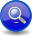#Boost C++ Libraries

...one of the most highly regarded and expertly designed C++ library projects in the world.

This is the documentation for an old version of Boost. Click here to view this page for the latest version.
##### Sequential Or Parser (```a || b```)
###### Description

The sequential-or operator, ```a || b```, matches `a` or `b` or `a` followed by `b`. That is, if both `a` and `b` match, it must be in sequence; this is equivalent to ```a >> -b | b```:

```a || b || ...
```
```// forwards to <boost/spirit/home/qi/operator/sequential_or.hpp>
#include <boost/spirit/include/qi_sequential_or.hpp>
```

Also, see Include Structure.

###### Model of

Notation

`a`, `b`
###### Expression Semantics

Semantics of an expression is defined only where it differs from, or is not defined in `NaryParser`.

Expression

Semantics

```a || b```

Match `a` or `b` in sequence. equivalent to ```a >> -b | b```

###### Attributes

Expression

Attribute

```a || b```

```a: A, b: B --> (a || b): tuple<optional<A>, optional<B> >
a: A, b: Unused --> (a || b): optional<A>
a: Unused, b: B --> (a || b): optional<B>
a: Unused, b: Unused --> (a || b): Unused

a: A, b: A --> (a || b): vector<optional<A> >```

NoteThe sequential-or parser behaves attribute-wise very similar to the plain sequence parser (```a >> b```) in the sense that it exposes the attributes of its elements separately. For instance, if you attach a semantic action to the whole sequential-or: ```(int_ || int_)[print_pair(_1, _2)] ``` the function object `print_pair` would be invoked with the attribute of the first `int_` (`boost::optional`) as its first parameter and the attribute of the second `int_` (`boost::optional` as well) as its second parameter.
###### Complexity

The overall complexity of the sequential-or parser is defined by the sum of the complexities of its elements. The complexity of the sequential-or itself is O(N), where N is the number of elements in the sequence.

###### Example
NoteThe test harness for the example(s) below is presented in the Basics Examples section.

Some using declarations:

```using boost::spirit::qi::int_;
```

Correctly parsing a number with optional fractional digits:

```test_parser("123.456", int_ || ('.' >> int_));  // full
test_parser("123", int_ || ('.' >> int_));      // just the whole number
test_parser(".456", int_ || ('.' >> int_));     // just the fraction
```

A naive but incorrect solution would try to do this using optionals (e.g.):

```int_ >> -('.' >> int_)  // will not match ".456"
-int_ >> ('.' >> int_)  // will not match "123"
-int_ >> -('.' >> int_) // will match empty strings! Ooops.
```
 Copyright © 2001-2011 Joel de Guzman, Hartmut Kaiser Distributed under the Boost Software License, Version 1.0. (See accompanying file LICENSE_1_0.txt or copy at http://www.boost.org/LICENSE_1_0.txt)